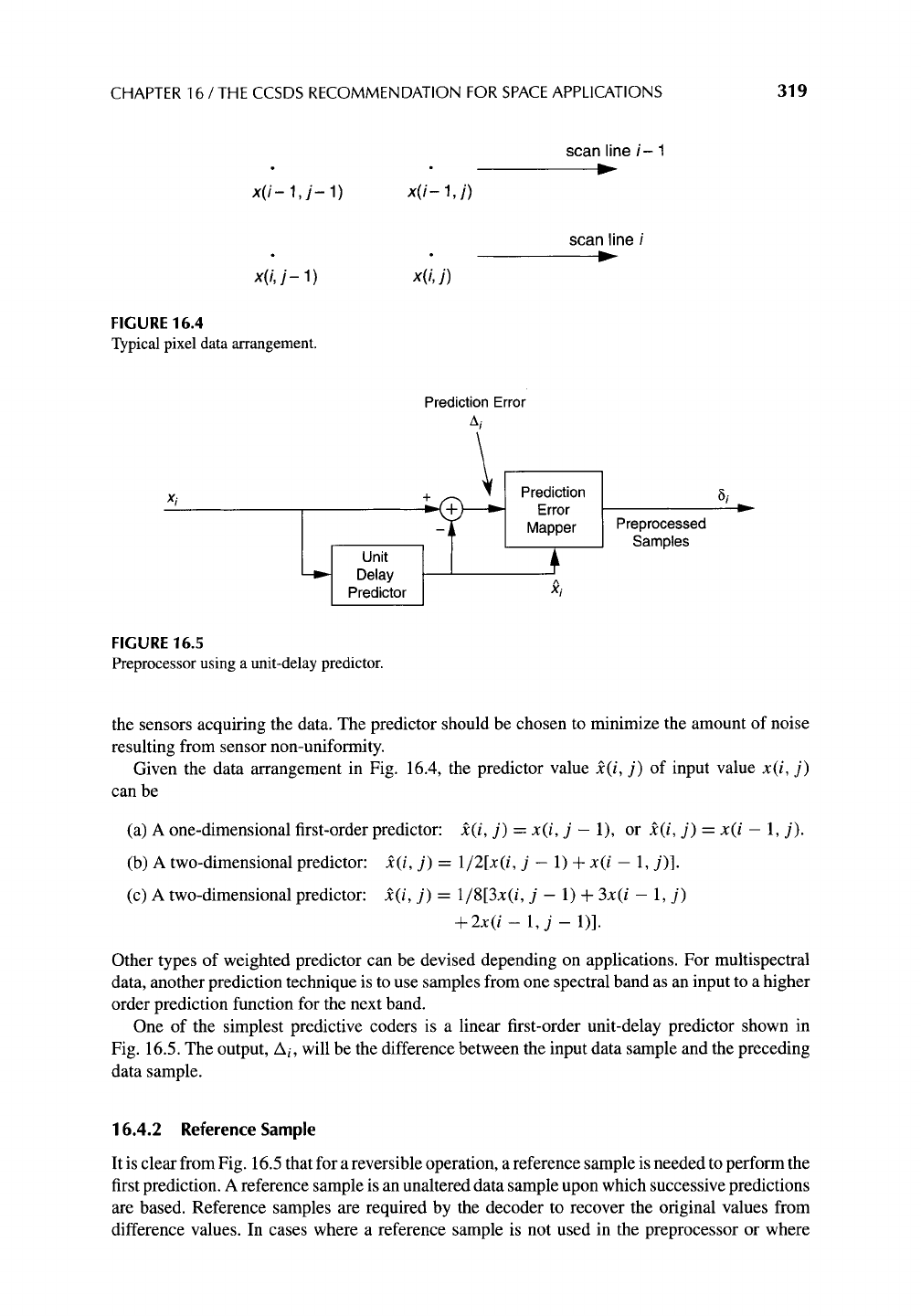318 PART IV / STANDARDS
Table 16.4 Selected Code Option Identification Key
Option n _< 8
8<n<16
16<n
u
Zero-Block 0000
Second-Extension 0001
FS 001
k -- 1 010
k=2 011
k = 3 100
k = 4 101
k=5 110
k=6
k---7
k--8
k=9 m
k=10
k=ll
k=12
k=13
k=14
k=15
9 o
9 o
k=29
No compression 111
00000 000000
00001 000001
0001 00001
0010 00010
0011 00011
0100 00100
0101 00101
0110 00110
0111 00111
1000 01000
1001 01001
1010 01010
1011 01011
1100 01100
1101 01101
1110 01110
01111
10000
11110
1111 11111
Note:
A dash indicates no applicable value.
16.4 PREPROCESSOR
The role of the preprocessor is to transform the data into samples that can be more efficiently
compressed by the entropy encoder. To ensure that compression is "lossless," the preprocessor
must be reversible. The FS and sample-split options work on non-negative integer values. The
most effective preprocessor will transform integer data values into all non,negative values with
a unimodal distribution resembling a one-sided Lapace function. The example in Section 16.3.1
shows that to achieve effective compression, we need a preprocessing stage that transforms the
original data so that shorter codewords occur with higher probability than longer codewords. If
there are several candidate preprocessing stages, we would like to select the one that produces
the shortest average codeword length.
In general a preprocessor that removes correlation between samples in the input data block
will improve the performance of the entropy coder. In the following discussion, we assume that
preprocessing is done by a predictor followed by a prediction error mapper. For some types of
data, more sophisticated transform-based techniques can offer improved compression efficiency,
at the expense of higher complexity.
16.4.1 Predictor
The decorrelation function of the preprocessor can be implemented by a judicious choice of
predictor for an expected set of data samples. The selection of a predictor should take into
account the expected data as well as possible variations in the background noise and the gain ofCHAPTER 16 / THE CCSDS RECOMMENDATION FOR SPACE APPLICATIONS 319
x(i- 1, j-
1)
x(i- 1, j)
x(i, j-
1)
x(i, j)
FIGURE 16.4
Typical pixel data arrangement.
scan line i- 1
scan line i
Prediction Error
Ai
Delay
A
Predictor
xi
FIGURE 16.5
Preprocessor using a unit-delay predictor.
Prediction
Error
Mapper
Preprocessed
Samples
8i
,,..._
the sensors acquiring the data. The predictor should be chosen to minimize the amount of noise
resulting from sensor non-uniformity.
Given the data arrangement in Fig. 16.4, the predictor value
2(i, j)
of input value
x(i, j)
can be
(a) A one-dimensional first-order predictor:
2(i, j) = x(i, j -
1), or
2(i, j) = x(i -
1, j).
(b) A two-dimensional predictor: ~(i, j) =
1/2[x(i, j -
1) +
x(i
- 1, j)].
(c) A two-dimensional predictor: ~(i, j) =
1/813x(i, j -
1) +
3x(i - 1, j)
+ 2x(i- 1, j-
1)].
Other types of weighted predictor can be devised depending on applications. For multispectral
data, another prediction technique is to use samples from one spectral band as an input to a higher
order prediction function for the next band.
One of the simplest predictive coders is a linear first-order unit-delay predictor shown in
Fig. 16.5. The output, Ai, will be the difference between the input data sample and the preceding
data sample.
16.4.2 Reference Sample
It is clear from Fig. 16.5 that for a reversible operation, a reference sample is needed to perform the
first prediction. A reference sample is an unaltered data sample upon which successive predictions
are based. Reference samples are required by the decoder to recover the original values from
difference values. In cases where a reference sample is not used in the preprocessor or where

Get Lossless Compression Handbook now with the O’Reilly learning platform.

O’Reilly members experience live online training, plus books, videos, and digital content from nearly 200 publishers.